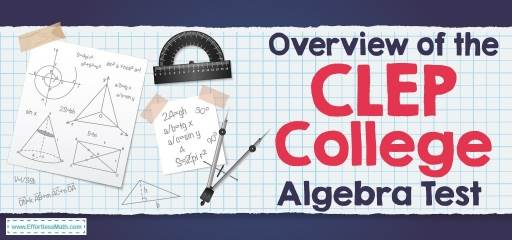# Overview of the CLEP College Algebra TestThe College Level Examination Program or CLEP offers 33 exams that cover college course content at the introductory level. The CLEP College Algebra exam lets students earn university credentials without having to attend classes. This test includes basic algebraic operations such as inequalities, linear and quadratic equations, and graphs. The CLEP College Algebra exam is computer-based, entirely multiple-choice questions. It contains 60 questions with a 90-minute time limit and includes pre-test questions that are not graded.

The College Algebra exam assesses test-takers abilities in the following things:

• Dissolve routine and straightforward problems (about 50% of the exam)
• Dissolve non-routine questions that require an understanding of concepts and application of concepts (about 50% of the exam)

## What topics are in the CLEP College Algebra test?

The subject of the College Algebra exam includes the following:

### Algebraic operations (25%)

• Operations with exponents
• Factoring and expanding polynomials
• Operations with algebraic expressions
• Absolute value
• Properties of logarithms

### Equations and inequalities (25%)

• Linear equations and inequalities
• Absolute value equations and inequalities
• Systems of equations and inequalities
• Exponential and logarithmic equations

### Functions and their properties (30%)

• Definition, interpretation, and representation/modeling (symbolic, graphical, numerical, and verbal)
• Domain and range
• Evaluation of functions
• Algebra of functions
• Graphs and their properties (including symmetry, intercepts, and transformations)
• Inverse functions

### Number systems and operations (20%)

• Real numbers
• Complex numbers
• Sequences and series
• Factorials and binomial theorem

## Is the CLEP College Algebra exam hard?

The CLEP College Algebra is not difficult and, you can get a good score by planning and enough effort.

## Do you get a formula sheet in the CLEP College Algebra exam?

You will not be given a formula sheet during the CLEP College Algebra test. It means that these formulas are easy, and you can easily memorize them.

## Can you use a calculator in the CLEP College Algebra exam?

You cannot bring your calculator but, a scientific calculator (without charts) is integrated into the exam software, and is available to you throughout the test.

## What is the passing score of the CLEP College Algebra exam?

This test is a score from 20 to 80 points, and a score of 50 is considered the minimum passing score.

Looking for the best resources to help you or your student succeed on the CLEP College Algebra test?

## The Best Books to Ace the CLEP College Algebra Test

### What people say about "Overview of the CLEP College Algebra Test - Effortless Math: We Help Students Learn to LOVE Mathematics"?

No one replied yet.

X
41% OFF

Limited time only!

Save Over 41%

SAVE \$10

It was \$24.99 now it is \$14.99